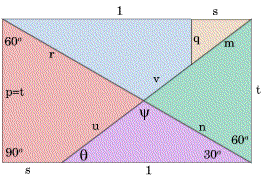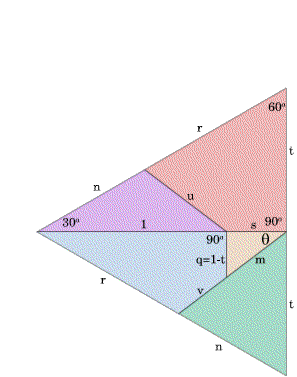#### You may also like### Degree Ceremony

What does Pythagoras' Theorem tell you about these angles: 90°, (45+x)° and (45-x)° in a triangle?### Logosquares

Ten squares form regular rings either with adjacent or opposite vertices touching. Calculate the inner and outer radii of the rings that surround the squares.### Ball Bearings

If a is the radius of the axle, b the radius of each ball-bearing, and c the radius of the hub, why does the number of ball bearings n determine the ratio c/a? Find a formula for c/a in terms of n.

# 30-60-90 Polypuzzle

##### Age 16 to 18 Challenge Level:

Finding the lengths depends on using the ratio for the sides of a 30-60-90 triangle as the name of the problem suggests. Below the diagrams show how to take the pieces which make a square of unit area and fit the pieces together to make an equilateral triangle of the same area with side $2t$ and knowing this you can calculate $t$. The way pieces fit together gives you that $p=t$ and the rest is up to you!

You can calculate the length '$t$' knowing the area of the equilateral triangle. Pythagoras theorem and the sine rule can be used in finding the other lengths.